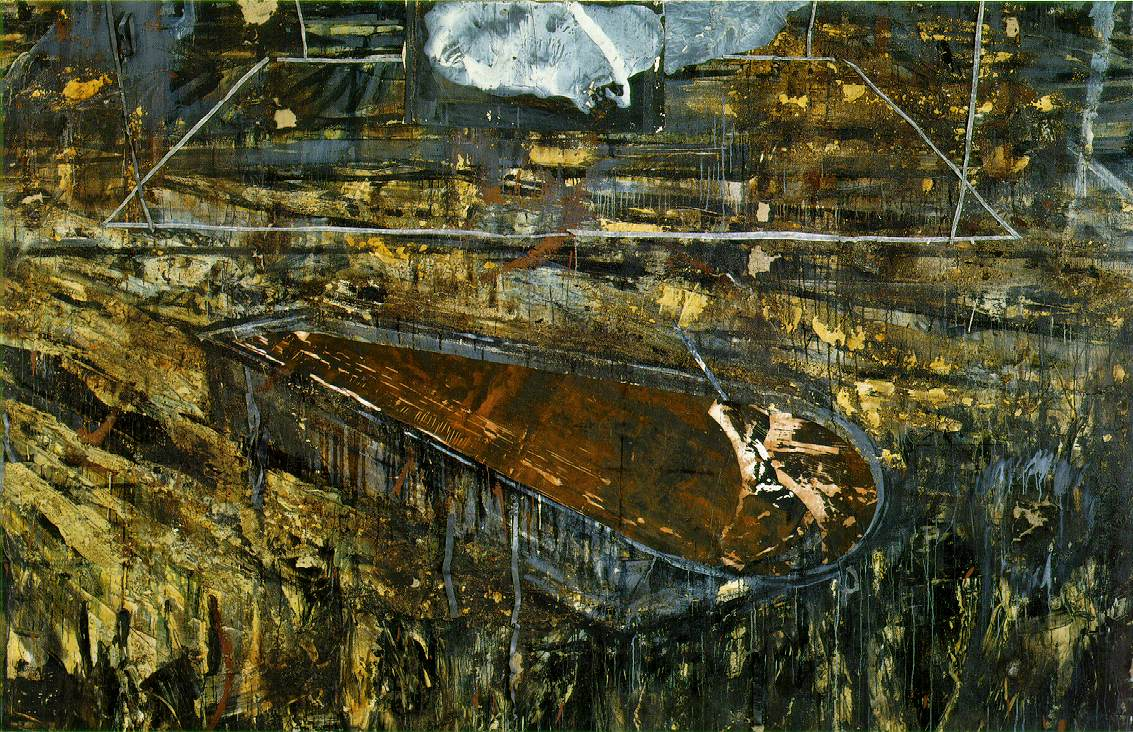# Exam 3 material

Sorry this is a bit late; this week’s been crazy (this whole year’s been crazy, ask the holy spirit to save me, only difference from me and Ossie Davis, gray hair maybe).

Come to my office hours tomorrow for help and to pick up your homework 8.
The solutions to homework 9 are now posted.

To do well on Exam 3 you should know all about the following:

– Linear independence
– The span of a set of vectors
– Subspaces
– col(A), row(A), null(A)
– rank(A)
– Linear transformations
– The condition number of a matrix and what means (Section 3.5)
– Linear regression (Section 4.2)
– The pseudoinverse of a matrix and what it is used for (Section 5.3)
Types of problems are:
– Determine whether a vector is in the span of some set of vectors
– Determine whether a set of vectors forms a basis for a space
– Prove some given set S is a subset of R^n, or finding a counterexample to show that it is not
– Prove that some given transformation T is linear, or finding a counterexample to show that it is not
– Find solution sets to underdetermined, consistent systems
– Find bases for col(A), row(A), null(A)
– Find the dimension of a space
– Find the rank of a matrix
– Given enough information about A to find its condition number without too much calculation, find cond(A). Given error matrix E, explain what cond(A) tells us about the possible error in our solution to the system Ax = b
– Find q in a regression model y^ = qx
– Find q for a more complicated model by converting data into values that are linearly related (as in section 4.2 #7, 8)
– Set up the regression problem y = qx + r as Xw = y and find least-squares solution w = [q, r] = (pseudoinverse(X)) * (y)
– Given enough information about A to find its pseudoinverse, find the least-squares solution w to Aw = b (the previous bullet point is a special case of this problem)
The best way to study is to redo the homework problems without using the textbook or your notes. Every problem on the exam is similar to some homework problem from the past few weeks.

Saw this painting in the MoMA last week, thought it was pretty dopeThe Red Sea, by Anselm Kiefer